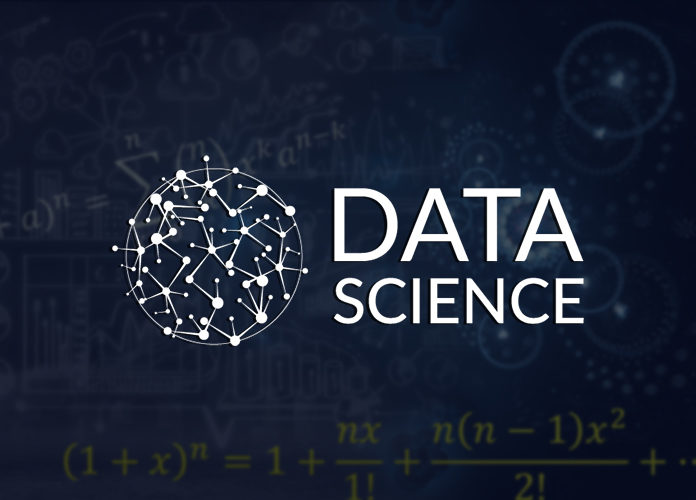# Statistics Primer for Data Science.

0
1043In Machine Learning, the core idea is to model the underlying phenomena that generates a particular data. For instance, when we try to predict the price of a house given parameters like carpet area in square feet, number of rooms, etc, we are essentially trying to develop a model that relates these parameters to the price of the house. Machine Learning helps in creating this model by taking a look at several sample data points. Simply speaking, Machine Learning tries to find the pattern between these parameters and the price of the house.

These patterns are generally statistical patterns. The patterns aren’t exactly perfect. For instance, even the best Machine Learning model may fail to accurately price the house. However, we can always get a confidence score as to how much our prediction is close to the actual value. This confidence score is measured generally in terms of probability. This is why it becomes important to learn Statistics to excel in Data Science.

Today we’re going to talk about certain important statistical concepts that are often used in Data Science.

Probability Theory

Probability of an event is defined as the likelihood that the event will occur. As a simple example, when we toss a fair coin, the likelihood that heads will show up is 0.5. Basically, there is a 50%-50% chance that a heads will come or a tail will show up. More formally, we say that P(Heads) = P(Tail) = 0.5.

Mathematically, probability of an event E is defined as the ratio of number of outcomes in the event E to the number of outcomes of sample space S.

P(E) = n(E)/n(S)

Here, S is the sample space – the space containing all possible outcomes. Let us take an example to understand it better. Suppose we roll a fair die. What is the probability that number 4 shows up?

Observe here that E is the event that number 4 shows up when a fair die is rolled. Also observe that the sample space, that is, the space of all events is {1, 2, 3, 4, 5, 6}. This indicates that we can have one of 1, 2, 3, 4, 5 and 6 that may show up.

So, the number of outcomes in event E is just 1 (when number 4 shows up). The number of outcomes in the sample space is 6 (when one of 1, 2, 3, 4, 5 or 6 shows up). So, n(E) = 1 and n(S) = 6 and so, P(E) = 1/6.

The concept of probability is central to Data Science and it forms the root of the most important concept in Data Science – concept of a Random Variable.

Random Variable

A random variable is a variable whose outcome is random and probabilistic. Let us understand better with an example. Consider the random variable X that denotes the number of heads obtained when a fair coin is tossed 3 times.

Here, we know that when a coin is tossed 3 times, the possible outcomes are: {(TTT), (TTH), (THT), (THH), (HTT), (HTH), (HHT), (HHH)}. Here, T indicates a tail and H indicates a head. So, THT means we got tail in the first toss, heads in the second and tail in the third toss.

The probabilities of these respective outcomes are as follows:

 Outcome Probability TTT 0.5 x 0.5 x 0.5 = 0.125 TTH 0.5 x 0.5 x 0.5 = 0.125 THT 0.5 x 0.5 x 0.5 = 0.125 THH 0.5 x 0.5 x 0.5 = 0.125 HTT 0.5 x 0.5 x 0.5 = 0.125 HTH 0.5 x 0.5 x 0.5 = 0.125 HHT 0.5 x 0.5 x 0.5 = 0.125 HHH 0.5 x 0.5 x 0.5 = 0.125

Let us get back to our random variable X that denotes the number of heads obtained.

 Outcome Number of heads (X) TTT 0 TTH 1 THT 1 THH 2 HTT 1 HTH 2 HHT 2 HHH 3

Clearly, the value of X is random and unpredictable in case of a fair coin. However, we can calculate the probability associated with each of the values that X takes. For instance, the probability that X takes the value 1, P(X = 1) can be calculated as:

P(X = 1) = P({TTH, THT, HTT}) / 8 = 3/8 = 0.375

Similarly, we can calculate other probabilities:

 Value of X Probability 0 1/8 = 0.125 1 3/8 = 0.375 2 3/8 = 0.375 3 1/8 = 0.125

So basically, a random variable can be defined by a “probability distribution function” like the one above where FX(x) = Probability that X takes the value x = P(X = x).

Depending on the complexity of FX(x), we can have several distribution functions. Let us take a look at some of the common examples.

## Statistics Primer Distribution Functions

The above example that we took was of a well-known distribution function called as a Binomial Distribution. Generalizing the above case – let Bn represents the number of heads obtained when we toss a fair coin n times. Clearly, Bn is a random variable since the number of heads obtained are probabilistic.

We can define the probability distribution function of Bn as P(Bn = k) which essentially denotes the probability that Bn takes the value ‘k’.

Of the n tosses, the number of ways of getting k heads is nothing but nCk. Now, for each of these ways, the probability is 1/2n. So, P(Bn = k) = nCk / 2n.

This defines the probability distribution function of a Binomial Distribution.

Just like Binomial Distribution, there are many other distribution functions. For instance, exponential distribution, poisson distribution, etc. Each of these distributions tries to model a specific underlying phenomena.

The concepts that we talked about in this blog are essential to understanding Statistical Data Modeling which is central to Data Science. It is recommended that you understand them thoroughly to develop a firm grasp of Data Science.